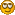###Author Topic: a pair function adapters for sort_array  (Read 2770 times)

####silenus

• BFF
•• Posts: 199##### a pair function adapters for sort_array
« on: April 17, 2007, 08:53:23 AM »
I wrote a pair of function adapters for sort array which came up in a problem in sorting and displaying list data. The first comparator() converts a binary "greater or equal than" comparison into the 3 value comparison needed by sort_array that returns 1,0, or -1 depending on if the value is greater, equal or less than the respective second value respectively for example to sort a list of integers with this function one would do the following:

sort_array(array_of_ints, comparator( (: \$1 >= \$2 :) ) )

The second function is for sorting tuples when you have to sort a list of items which consist of lists of data or tuples where each element at a given index is of the same type. i.e. ({"Silenus", 1, "orc", "warrior"}), ({"Cratylus", 1, "human", "mage"}) it creates a comparison function which gives priority to the indexes as ordered. An example usage might be (using the previous data)-

sort_array(array_of_tuples, tuple_comparator( ({ comparator( (: \$1 >= \$2 :) ), (: strcmp(\$1,\$2) :) }), ({1,0}) ) )

which prioritizes the sort by level and if the levels are found to be equal sorts by name.

Both functions rely on tricks from functional programming in that they both create functions on the fly based on a function or functions passed in. The code might be a bit cryptic to those not use to this style but is illustrative of this approach.

/* Converts a greator or equal binary comparator into a 3 value comparator */
public function comparator(function f)
{
return (: evaluate(\$(f), \$1, \$2) ? ( evaluate(\$(f), \$2, \$1) ? 0 : 1) : -1 :);
}

/*
Compares two tuples in the order specified by the indices. If a comparator
applied to an index early in the order results in equality, the next
comparator is used on the next index on the list.
*/
private function tuple_comparator_(function array, int array);

public function tuple_comparator(function array f, int array i)
{
if( sizeof(f) != sizeof(i) ) error("Argument arrays must be of the same size.");
return tuple_comparator_(f,i);
}

private function tuple_comparator_(function array f,int array i)
{
if( sizeof(f) == 0 ) return (: 0 :);
return (: evaluate( \$(f), \$1[\$(i)], \$2[\$(i)] ) != 0 ? evaluate( \$(f), \$1[\$(i)], \$2[\$(i)] ) : evaluate( \$(tuple_comparator_(f[1..],i[1..])), \$1, \$2 ) :);
}

####saquivor##### Re: a pair function adapters for sort_array
« Reply #1 on: April 17, 2007, 01:00:35 PM »
Looks like great code.

Could you please post a practical example of how this may be used. As it may help others (including me) to undertand the code even better and see how they would plug it in to their mud.

Thanks,
Saquivor.

####silenus

• BFF
•• Posts: 199##### Re: a pair function adapters for sort_array
« Reply #2 on: April 18, 2007, 04:33:06 AM »
I see those more as library type functions which you can use to help you sort objects if you need to. One possible application is perhaps in something like the who code where you need to sort something in blocks. I posted them more to illustrate the technique more than anything else. Here's maybe a brief example using DS style function calls-

Code: [Select]
`// sorts array according to race then class then level then name...mixed array who_sort(){    mixed array ar = map( users(), (: ({\$1->GetName(), \$1->GetLevel(), \$1->GetRace(), \$1->GetClass() }) :) );      function f = tuple_comparator(         ({ (: strcmp(\$1,\$2) :), (: strcmp(\$1,\$2) :), comparator( (: \$1 >= \$2 :) ), (: strcmp(\$1,\$2) :) }),              ({2,3,1,0}) );    return sort_array(ar, f);}`